# How does QUANTUM MECHANICS turn into CLASSICAL MECHANICS?

## 1. Solution of Schrodinger's Equation

The Schrodinger Equation for the wavefunction of a particle with Hamiltonian H is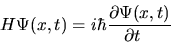This can be solved by separation of variables. Consider a separable solution: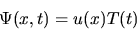The separated functions satisfy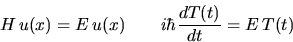The equation for u is the time-independent Schrodinger equation, and is also
the eigenvalue equation for H, where E is the eigenvalue.
It has a set of solutions un(x) with eigenvalues En.
The equation for T has the solution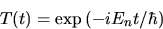We therefore obtain a set of separated solutions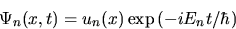Because of the linearity of the Schrodinger equation any sum of these with
complex constant coefficients is also a solution.
The general solution of the Time Dependent Schrodinger Equation
(with a Hamiltonian independent of time) is thus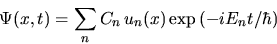## 2. Getting Information from the Wavefunction

The wavefunction contains our knowledge of the system at time t, and using the
standard methods of quantum mechanics we can obtain a probability distribution for
the result of any measurement at any time. For example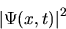is the probability distribution for the position x of the particle at time t.
We can then find the mean and standard deviation of this probability distribution: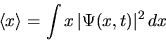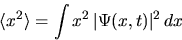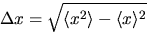The corresponding results for momentum p are: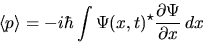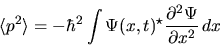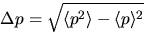Delta x and Delta p are constrained by the uncertainty principle: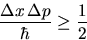## 3. The Classical Limit

Classical Mechanics predicts a unique x(t), p(t), from precise initial data x(0), p(0).
In the classical limit of quantum mechanics we would like to see:

• A compact probability distribution (a wavepacket);
• A `small' value of Delta x;
• A `small' value of Delta p;
• Time evolution such that <x(t)> and <p(t)>
follow the classical x(t) and p(t).
We cannot achieve this with single eigenfunctions because the space part un(x) is never compact:
in fact it always fills the classically available space (i.e. where the potential energy V(x)
is less than the energy eigenvalue En). Furthermore, the expectation values for a single eigenfunction
are time-independent (that's why it's called a stationary state).
The classical limit necessarily involves superposition.

In fact quite a modest superposition of 20 states produces quite a compact wavefunction, and reduces
the Delta x Delta p product to close to the uncertainty principle limit.
The Wavepacket Plotter illustrates the time-evolution of this wavepacket solution in a 1-dimensional box.

## 4. Wavepacket Motion in a 1-dimensional Box

The infinite square well is a simple model of a one-dimensional box of width a: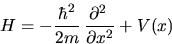The potential energy function V(x) is given by
V(x)=0 for 0 < x < a and V(x)=infinity for x < 0 and x > a.

Any quantum mechanics textbook will show that the eigenvalues and eigenfunctions are given by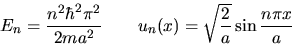The Wavepacket Plotter generates a wavepacket using eigenfunctions with quantum numbers centred from nmin to nmax.
THE OLD APPLET HASBEEN REPLAXED BY RGE WAVEFUNCTION PLOTTER. THE TEXT HERE NEDS UPDATING

The complex constant coefficients Cn are given by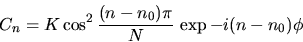where N = 2dn + 2 and K ensures that the wavefunction is properly normalised.
(There is no particular reason for this form, except that it is a `smooth' function of n.)

The phase phi controls where the wavepacket is at t = 0.
The default value is pi/2, entered in degrees as 90., which produces a packet in the centre of the box
moving in the positive direction at t = 0.

#### Units

The Applet uses units such that the mass of the particle is 1 mass unit, and the width of box is
1 length unit. The time unit is set such that the classical round trip time in the box for the
particle in quantum state n0 is 1 time unit. In these units Planck's constant over two pi
(hbar) has the value 2/pi n0, so another way of viewing these units is that we are simulating
the effect on a fixed particle in a box of changing the size of hbar: the default settings change
hbar from 10-34 SI units to 0.006 Applet units!

## 5. Suggestions for Applet Use

#### Getting Started

When the Applet is started it will display the position probability distribution at t=0.
Check that it looks roughly normalised (height times width is about one).
Check that the <x> and Delta x values in the text panel correspond with the plot.

Look at the Cn, Un and En plots to make sure you understand how the wavepacket
is made up. The En plot is part of a parabola - you should just about see the curvature.

Now return to the |Psi(x)|2 plot and note the value of <p>.
Click on Increment t button, and check that <x> has increased by about <p>/64
(Remember that the mass is one, so <p> is effectively the velocity, and the time increment is 1/64.)

Now click on the Increment t button several times till the wavepacket reaches the wall of the box.
Notice that <x(t)> increases very slightly, but at the turn-around it suddenly decreases.
Can you work out why? And what's happened to the probability distribution?

Now go a bit further forward in time and the wavepacket will go into reverse.
What's happened to <x> and <p> now?

Reset the time to -4.0 and change the plot to <x> vs t. (This may take a bit of time).
You will now see the wavepacket position and width compared to the classical limit.
(The classical prediction is based on <p>=2 exactly.)
Notice that the packet is sharply focussed around t=0 and is broader at earlier and later times.
Change back to |Psi(x)|2 and t=8. Notice that the packet fills the box
and Delta x is close to 1/sqrt(12)=0.289, which corresponds complete ignorance of its position.
At later times the <x> vs t plot shows very little oscillation in <x>,
and Delta x very close to complete ignorance.

So the wavepacket represents a fairly classical particle oscillating in the box, with our
uncertain knowledge of its initial data leading to an increasing uncertainty of position at later times.

#### Some Ideas for Further Study

Look at the effect of varying the phase on the position of the particle at t=0.
Is the wavepacket with t=0 and phase=135 the same as t=0.125 and phase=90?
How does the width of the wavepacket at t=0 vary with n and dn? What about the time it stays focussed for?

The spreading of the wavepacket is obvious in classical terms - can you explain it in
terms of the wavefunction? (Think about how the phase variable controls the position of the packet.)

Now for a surprise! Look at the wavepacket at t=108. (or whatever is the current value of n0).
Now how did that happen? Look at <x> vs t around this region.
In fact the solution is exactly periodic with period n0. This re-focussing is called a revival.